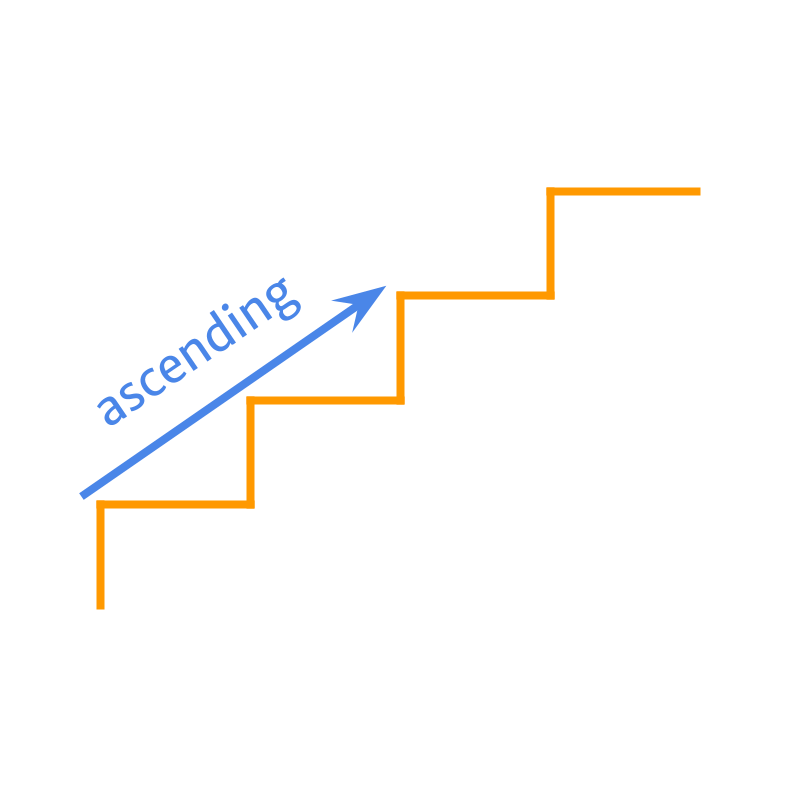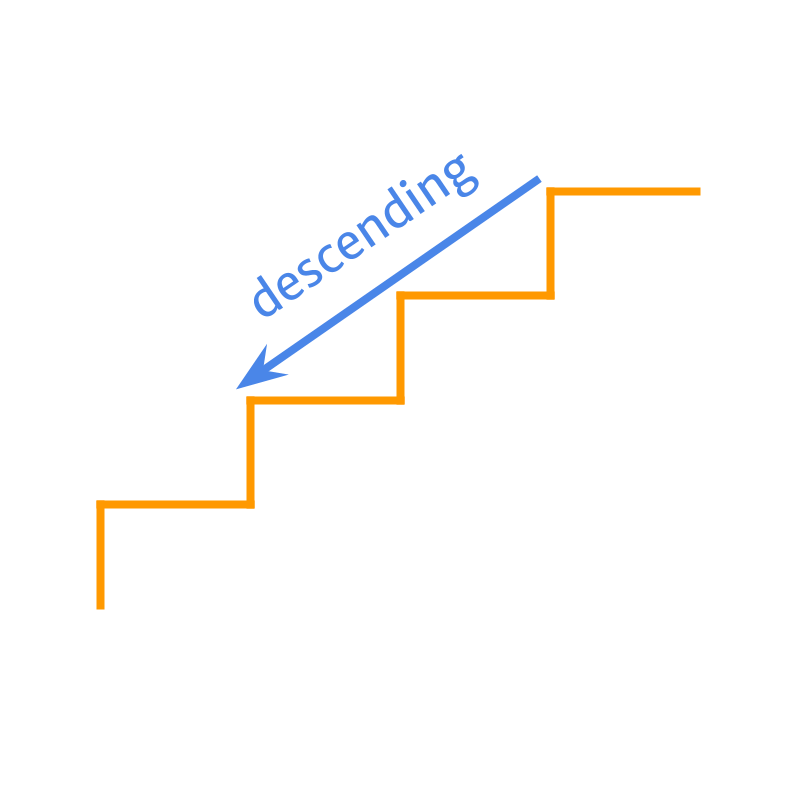maths > integers

Ascending and Descending Orders in Integers

what you'll learn...

overview

This page extends the comparison of integers to arranging three or more numbers in ascending or descending order.

order in manyThe ascending order was introduced in whole numbers. Given numbers are arranged from smallest to largest.The descending order was introduced in whole numbers. Given numbers are arranged from largest to smallest.

Arrange 234$- 234$, 423$423$, 73$73$ in ascending order.

The order is 234$- 234$, 73$73$, 423$423$.

Arrange $9$, $873$, $- 99$ in descending order.

The order is $873$, $9$, $- 99$.

Two or more integers can be compared to arrange them in
•  ascending order : from the smallest to the largest
•  descending order : from the largest to the smallest

examples

Arrange the numbers in ascending order $- 8$, $- 843$, $- 84$.
The answer is "$- 843$, $- 84$, $- 8$"

Arrange the numbers in descending order $- 2$, $7$, $- 4$.
The answer is "$7$, $- 2$, $- 4$"

Arrange the numbers in descending order $5$, $- 5$, $5$, $- 5$.
The answer is "$5$, $5$, $- 5$, $- 5$"

Arrange the numbers in ascending order $- 2$, $0$, $4$.
The answer is "$- 2 , 0 , 4$"

summary

More than two numbers can be compared to arrange them in

•  ascending order : from smallest to the largest•  descending order : from largest to the smallestExample: given numbers $2 , 7 , - 4 , - 6$ is arranged in
ascending order : $- 6 , - 4 , 2 , 7$
descending order : $7 , 2 , - 4 , - 6$

Outline